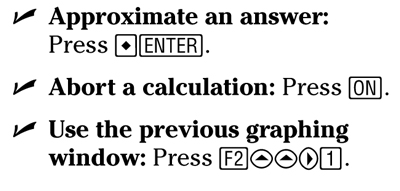##### TI-89 Graphing Calculator For DummiesYour TI-89 graphing calculator (along with the TI-89 Titanium, TI-92 Plus, and Voyage 200) help you graph and so much more. You can do higher math functions, include symbols, and format equations as well as make use of the basic calculator functions — and some odd ones, too!

## How to find higher math functions on the TI-89 graphing calculator

Of course, your TI-89 graphing calculator helps you with higher math functions — that’s part of the reason you bought it. However, you might need help finding everything. The following list doesn’t tell you how to locate everything, but it does show you how to get to some of the buttons that help you do higher math calculations: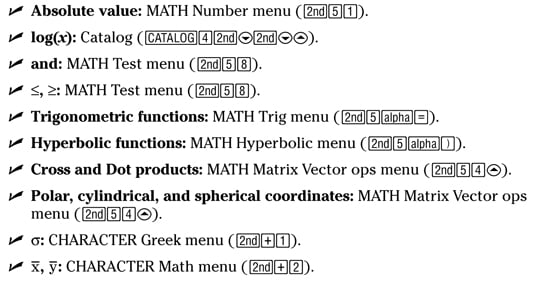## How to format equations on the TI-89 graphing calculator

Your TI-89 graphing calculator can help you solve equations and systems of equations. All you have to do is put them in the proper format. Fortunately, the following list shows how to format equations so that the TI-89 graphing calculator can help solve them.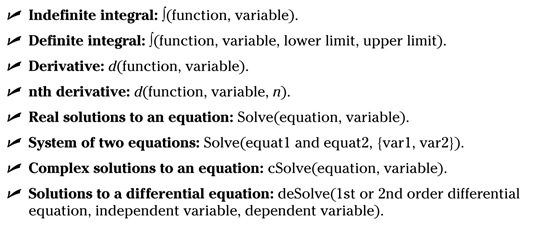## Special keys on the TI-89 graphing calculator

The TI-89 graphing calculator has so many functions, you may have trouble locating them all. But if you’re looking for theta or infinity (and who isn’t, right?), look no further than the following list, which shows you where to find those and some other more common functions: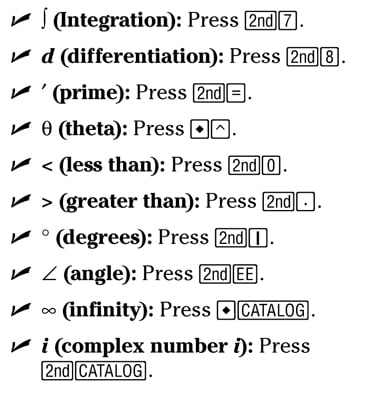## Basic keystrokes on the TI-92 and Voyage 200 graphing calculators

The TI-89, TI-92, and Voyage 200 calculators have similar functions, but in the case of the TI-92 and Voyage 200, different ways of accessing them. The following list shows how to access some basic functions on the TI-92 and Voyage 200.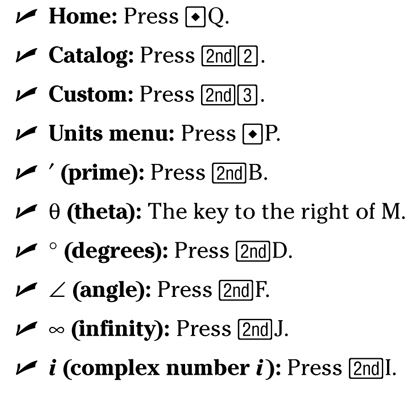## How to access symbols on the TI-89 graphing calculator

Of course your calculator needs a not-equal-to sign, but the TI-89 graphing calculator also offers the @, an exclamation mark, and a sigma. The following list shows you how to produce these symbols and more: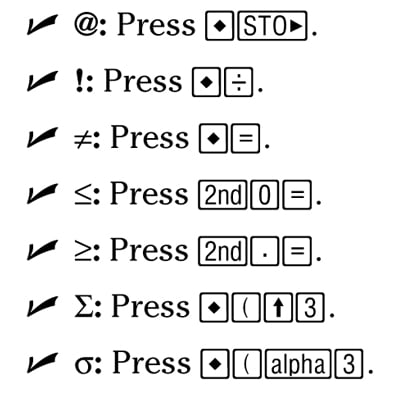## Accessing odd functions on the TI-89 graphing calculator

The TI-89 graphing calculator has all kinds of special functions. It can, of course, give you the mathematically certain answer you need, but it can also offer an approximation. You can tell it to stop calculating if need be, and you can go back to a previous graph. The following short list shows you how to do each: